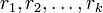# Criterion for element of alternating group to be real

(diff) ← Older revision | Latest revision (diff) | Newer revision → (diff)
Suppose$n$ is a natural number and$A_n$ is the Alternating group (?) on a set of size$n$. An element$g \in A_n$ is a Real element (?) if and only if the cycle decomposition of$g$ satisfies one of these three conditions:
3. All the cycles have distinct odd lengths$r_1,r_2,\dots,r_k$ are all distinct and$\sum_{i=1}^k (r_i - 1)/2$ is even.
Note that the permutations which satisfy condition (1) or (2) are precisely those whose conjugacy class is unsplit from the symmetric group, where every element is real. (see splitting criterion for conjugacy classes in the alternating group). (3) is the case of a conjugacy class that splits in$A_n$ but each element still remains with its inverse.